How to Convert Number to Words in Excel - Compute Expert

# How to Convert Number to Words in Excel

Home >> Excel Tutorials from Compute Expert >> Excel Tips and Trick >> How to Convert Number to Words in Excel

In this tutorial, you will learn completely how to convert a number to words in excel.

When processing numbers in excel, especially if they are financial related numbers, we sometimes need the numbers’ words form. Unfortunately, there isn’t a built-in excel formula that can help us to convert our numbers to their word form directly. However, there are some alternative methods we can implement which will give us similar results.

Want to know what are those methods in excel to convert our numbers to words properly? Read all parts of this tutorial.

Disclaimer: This post may contain affiliate links from which we earn commission from qualifying purchases/actions at no additional cost for you. Learn more

## Why We Need to Learn About Excel Convert Number to Words?

If the numbers you want to convert to words are small numbers, then you can use VLOOKUP to do it.

However, before you write the VLOOKUP, you need to create a table that consists of numbers and their word forms. The table will then become the reference where VLOOKUP will find the word form of your number.

Generally, the writing form of your VLOOKUP for this number conversion process will become like this.

= VLOOKUP ( number , reference_table , word_form_col_index , FALSE )

We input our number as the lookup reference value and the column index of the words form in our reference table. We input FALSE as the VLOOKUP search mode because we want the exact word form for our number (if we input TRUE, then VLOOKUP may take the wrong word form for our number because of its approximate search mode).

To better understand the VLOOKUP method implementation, see the example below.

Let’s say we want to convert this number into words.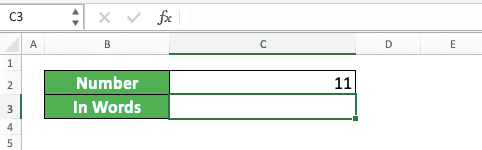How to do the conversion? Using the VLOOKUP method, we need to create a number-words table first as the VLOOKUP reference table.

To make the display cleaner, it is better if we create the table on a separate sheet. For the example, we create a reference table like this for our VLOOKUP.If you need to, you can create a much bigger reference table to be able to convert more numbers!

After you have the table ready, just write VLOOKUP in the cell where you want the words form of your number. Input the number you want to convert, the reference table, the words column index, and FALSE to the VLOOKUP.

Here is the VLOOKUP writing to convert the number in the example.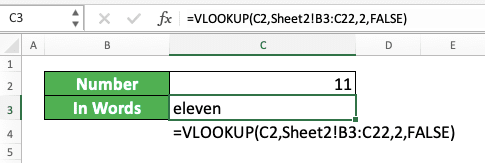As you have already created the reference table, you can use it later when you have other numbers to convert!

Admittedly, this method can only convert a small selection of numbers. What if you have numbers with the value of millions or more? If that is the case, then you should use one of the two methods we will discuss next instead.

## How to Convert Number to Words in Excel 2: Various Formulas Combination

Using multiple formulas in one writing, we can also convert our number to its word form in excel. The formula writing, however, is quite long so you might need to copy the formula from here instead of writing it yourself :)

Just so you know, we don’t write the formula we will show below ourselves. The credit all goes to an Excel Forum user with the Id HaroonSid who managed to get this gem out. We edit the formula a little bit so it will translate a number into pure words without currency terms.

Here is the formula that HaroonSid has formulated to solve the number to words conversion problem in excel. This assumes your number is in the B2 cell. Therefore, you need to change the B2 cell coordinate there if you put your number in another cell.

=IF(OR(LEN(FLOOR(B2,1))=13,FLOOR(B2,1)<=0),"Out of range",PROPER(SUBSTITUTE(CONCATENATE(CHOOSE(MID(TEXT(INT(B2),REPT(0,12)),1,1)+1,"","one hundred ","two hundred ","three hundred ","four hundred ","five hundred ","six hundred ","seven hundred ","eight hundred ","nine hundred "),CHOOSE(MID(TEXT(INT(B2),REPT(0,12)),2,1)+1,"",CHOOSE(MID(TEXT(INT(B2),REPT(0,12)),3,1)+1,"ten","eleven","twelve","thirteen","fourteen","fifteen","sixteen","seventeen","eighteen","nineteen"),"twenty","thirty","forty","fifty","sixty","seventy","eighty","ninety"),IF(VALUE(MID(TEXT(INT(B2),REPT(0,12)),2,1))>1,CHOOSE(MID(TEXT(INT(B2),REPT(0,12)),3,1)+1,"","-one","-two","-three","-four","-five","-six","-seven","-eight","-nine"),IF(VALUE(MID(TEXT(INT(B2),REPT(0,12)),2,1))=0,CHOOSE(MID(TEXT(INT(B2),REPT(0,12)),3,1)+1,"","one","two","three","four","five","six","seven","eight","nine"),"")),IF(B2>=10^9," billion ",""),CHOOSE(MID(TEXT(INT(B2),REPT(0,12)),4,1)+1,"","one hundred ","two hundred ","three hundred ","four hundred ","five hundred ","six hundred ","seven hundred ","eight hundred ","nine hundred "),CHOOSE(MID(TEXT(INT(B2),REPT(0,12)),5,1)+1,"",CHOOSE(MID(TEXT(INT(B2),REPT(0,12)),6,1)+1,"ten","eleven","twelve","thirteen","fourteen","fifteen","sixteen","seventeen","eighteen","nineteen"),"twenty","thirty","forty","fifty","sixty","seventy","eighty","ninety"),IF(VALUE(MID(TEXT(INT(B2),REPT(0,12)),5,1))>1,CHOOSE(MID(TEXT(INT(B2),REPT(0,12)),6,1)+1,"","-one","-two","-three","-four","-five","-six","-seven","-eight","-nine"),IF(VALUE(MID(TEXT(INT(B2),REPT(0,12)),5,1))=0,CHOOSE(MID(TEXT(INT(B2),REPT(0,12)),6,1)+1,"","one","two","three","four","five","six","seven","eight","nine"),"")),IF(VALUE(MID(TEXT(INT(B2),REPT(0,12)),4,3))>0," million ",""),CHOOSE(MID(TEXT(INT(B2),REPT(0,12)),7,1)+1,"","one hundred ","two hundred ","three hundred ","four hundred ","five hundred ","six hundred ","seven hundred ","eight hundred ","nine hundred "),CHOOSE(MID(TEXT(INT(B2),REPT(0,12)),8,1)+1,"",CHOOSE(MID(TEXT(INT(B2),REPT(0,12)),9,1)+1,"ten","eleven","twelve","thirteen","fourteen","fifteen","sixteen","seventeen","eighteen","nineteen"),"twenty","thirty","forty","fifty","sixty","seventy","eighty","ninety"),IF(VALUE(MID(TEXT(INT(B2),REPT(0,12)),8,1))>1,CHOOSE(MID(TEXT(INT(B2),REPT(0,12)),9,1)+1,"","-one","-two","-three","-four","-five","-six","-seven","-eight","-nine"),IF(VALUE(MID(TEXT(INT(B2),REPT(0,12)),8,1))=0,CHOOSE(MID(TEXT(INT(B2),REPT(0,12)),9,1)+1,"","one","two","three","four","five","six","seven","eight","nine"),"")),IF(VALUE(MID(TEXT(INT(B2),REPT(0,12)),7,3))," thousand ",""),CHOOSE(MID(TEXT(INT(B2),REPT(0,12)),10,1)+1,"","one hundred ","two hundred ","three hundred ","four hundred ","five hundred ","six hundred ","seven hundred ","eight hundred ","nine hundred "),CHOOSE(MID(TEXT(INT(B2),REPT(0,12)),11,1)+1,"",CHOOSE(MID(TEXT(INT(B2),REPT(0,12)),12,1)+1,"ten","eleven","twelve","thirteen","fourteen","fifteen","sixteen","seventeen","eighteen","nineteen"),"twenty","thirty","forty","fifty","sixty","seventy","eighty","ninety"),IF(VALUE(MID(TEXT(INT(B2),REPT(0,12)),11,1))>1,CHOOSE(MID(TEXT(INT(B2),REPT(0,12)),12,1)+1,"","-one","-two","-three","-four","-five","-six","-seven","-eight","-nine"),IF(VALUE(MID(TEXT(INT(B2),REPT(0,12)),11,1))=0,CHOOSE(MID(TEXT(INT(B2),REPT(0,12)),12,1)+1,"","one","two","three","four","five","six","seven","eight","nine"),""))),"  "," ")&IF(FLOOR(B2,1)>1,"",""))&IF(ISERROR(FIND(".",B2,1)),""," "&PROPER(IF(LEN(LEFT(TRIM(MID(SUBSTITUTE(Sheet1!B2,".",REPT(" ",255)),255,200)),2))=1,CHOOSE(1*LEFT(TRIM(MID(SUBSTITUTE(Sheet1!B2,".",REPT(" ",255)),255,200)),2),"ten","twenty","thirty","forty","fifty","sixty","seventy","eighty","ninety")&"","")&CONCATENATE(CHOOSE(MID(TEXT(INT(LEFT(TRIM(MID(SUBSTITUTE(Sheet1!B2,".",REPT(" ",255)),255,200)),2)),REPT(0,12)),11,1)+1,"",CHOOSE(MID(TEXT(INT(LEFT(TRIM(MID(SUBSTITUTE(Sheet1!B2,".",REPT(" ",255)),255,200)),2)),REPT(0,12)),12,1)+1,"ten","eleven","twelve","thirteen","fourteen","fifteen","sixteen","seventeen","eighteen","nineteen")&"","twenty","thirty","forty","fifty","sixty","seventy","eighty","ninety"),IF(VALUE(MID(TEXT(INT(LEFT(TRIM(MID(SUBSTITUTE(Sheet1!B2,".",REPT(" ",255)),255,200)),2)),REPT(0,12)),11,1))>1,CHOOSE(MID(TEXT(INT(LEFT(TRIM(MID(SUBSTITUTE(Sheet1!B2,".",REPT(" ",255)),255,200)),2)),REPT(0,12)),12,1)+1,"","-one","-two","-three","-four","-five","-six","-seven","-eight","-nine")&"",IF(LEFT(TRIM(MID(SUBSTITUTE(Sheet1!B2,".",REPT(" ",255)),255,200)),2)="01","one cent",IF(LEFT(TRIM(MID(SUBSTITUTE(Sheet1!B2,".",REPT(" ",255)),255,200)),1)="0",CHOOSE(MID(TEXT(INT(LEFT(TRIM(MID(SUBSTITUTE(Sheet1!B2,".",REPT(" ",255)),255,200)),2)),REPT(0,12)),12,1)+1,"","one","two","three","four","five","six","seven","eight","nine")&"","")))))))

Here, what we can tell is the formula uses CHOOSE as its core to converts the number in B2 to words. Mostly, it convert the number writing format using the TEXT formula first before separating its parts using MID.

From the MID result, CHOOSE will determine the correct word form for the part of the number. It uses the logic with other formulas help like SUBSTITUTE and IF to convert each part of our number into words.

For the implementation example of the formula writing, take a look at the screenshot below.As you can see, we manage to convert our number to words using the formula!

## How to Convert Number to Words in Excel 3: Custom Formula (VBA)

If you don’t use the two methods we have already discussed, then there is one more method you can try. For this method, we use VBA code to create a custom formula that can convert our numbers into words easily.

To use this method, however, you need to enable Macro first in your excel workbook so we can use VBA. From there, you must copy the VBA code we have prepared below to your workbook before you can utilize the formula.

To make it easier for you, we divide the discussion for this method into three parts. Those parts are the macro enablement, the VBA code copy process, and the usage of the formula. We will discuss each part in a step-by-step style.

### Enable Macro by Checking and Setting Macro Security Setting

To be able to use a custom formula from a VBA code in excel, you need to permit macro. Therefore, we need to ensure its correct permission setting first by doing the following steps.

1. Click File from the menu tab (In Mac, click Excel on the top left of your screen)2. Click Options (In Mac, click Preferences)3. Click Trust Center (In Mac, click Security. After that, please proceed to step 6)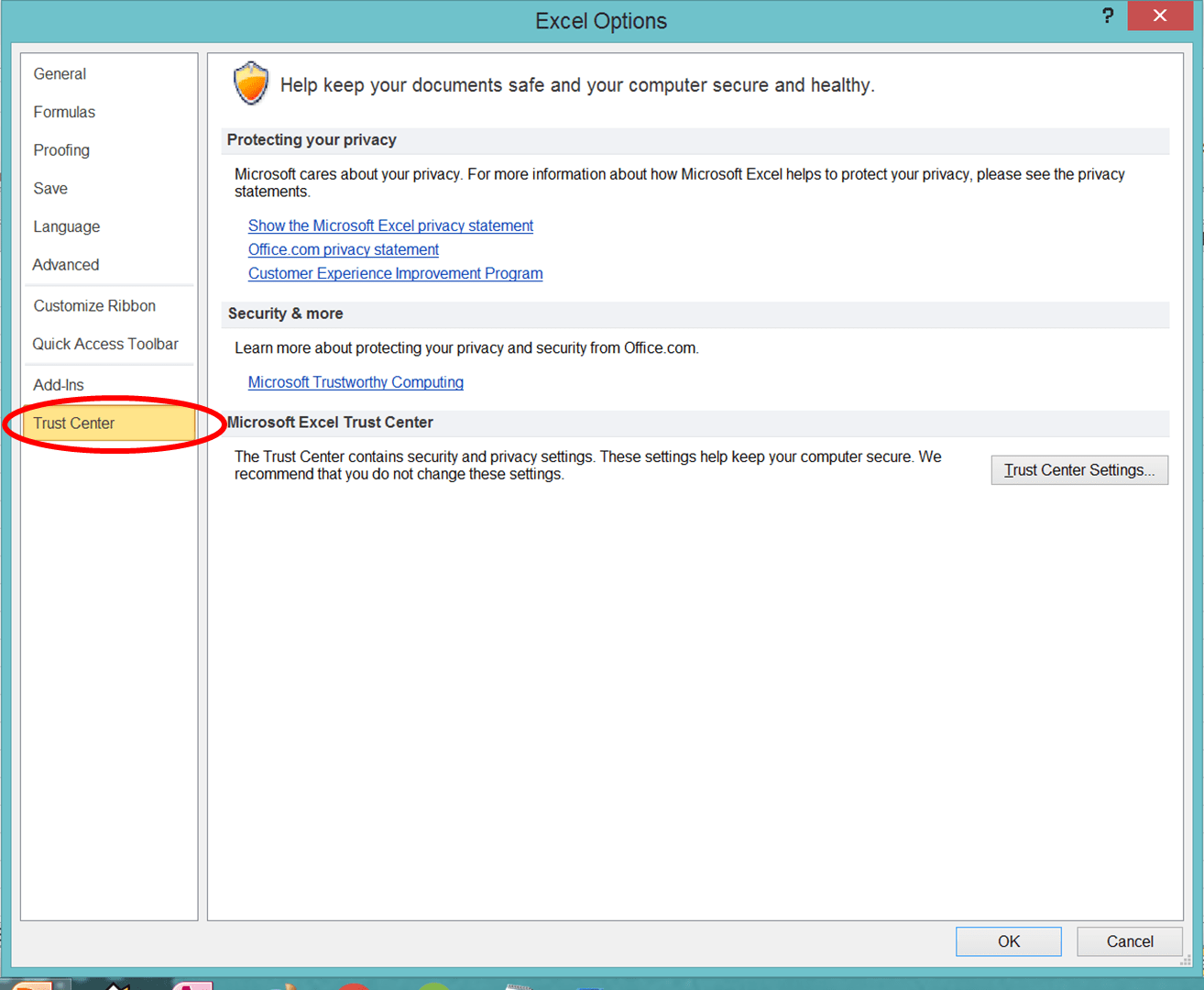4. Click Trust Center Settings...5. Click Macro Settings6. Choose Enable All Macros7. Click OK and OK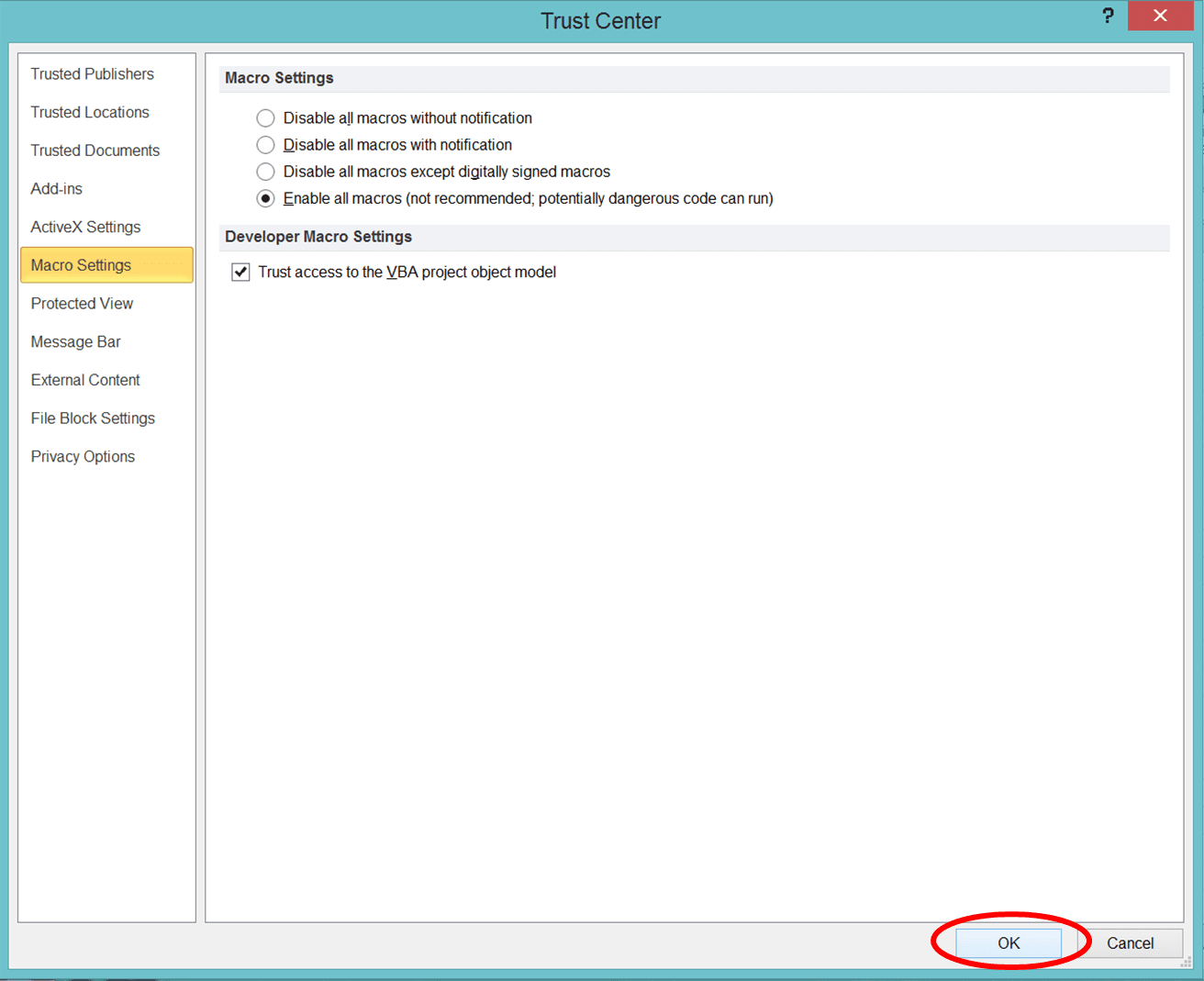8. Click File and then Save As9. On the Save as Type: dropdown, choose Excel Macro-Enabled Workbook10. Name your file in the File Name: text box11. Click Save### Create the Custom Formula to Convert Number to Words in Excel Using VBA Code

After setting our macro permission, it is time to create the formula using VBA.

1. Press Alt and F11 (Option + F11 atau Option + Fn + F11 di Mac) at the same time on your keyboard
2. Right-click on “VBA Project…”3. Highlight Insert with your pointer and click Module4. Double Click on the Module that comes up. Paste this code on the right side of the VBA editor screenFunction NUMBERTOTEXT(ByVal n As Currency) As String

Const Thousand = 1000@
Const Million = Thousand * Thousand
Const Billion = Thousand * Million
Const Trillion = Thousand * Billion

If (n = 0@) Then NUMBERTOTEXT = "zero": Exit Function

Dim Buf As String: If (n < 0@) Then Buf = "negative " Else Buf = ""
Dim Frac As Currency: Frac = Abs(n - Fix(n))
If (n < 0@ Or Frac <> 0@) Then n = Abs(Fix(n))
Dim AtLeastOne As Integer: AtLeastOne = n >= 1

If (n >= Trillion) Then
Buf = Buf & EnglishDigitGroup(Int(n / Trillion)) & " trillion"
n = n - Int(n / Trillion) * Trillion
If (n >= 1@) Then Buf = Buf & " "
End If

If (n >= Billion) Then
Buf = Buf & EnglishDigitGroup(Int(n / Billion)) & " billion"
n = n - Int(n / Billion) * Billion
If (n >= 1@) Then Buf = Buf & " "
End If

If (n >= Million) Then
Buf = Buf & EnglishDigitGroup(n \ Million) & " million"
n = n Mod Million
If (n >= 1@) Then Buf = Buf & " "
End If

If (n >= Thousand) Then
Buf = Buf & EnglishDigitGroup(n \ Thousand) & " thousand"
n = n Mod Thousand
If (n >= 1@) Then Buf = Buf & " "
End If

If (n >= 1@) Then
Buf = Buf & EnglishDigitGroup(n)
End If

If (Frac = 0@) Then
Buf = Buf
ElseIf (Int(Frac * 100@) = Frac * 100@) Then
If AtLeastOne Then Buf = Buf & " "
Buf = Buf & Format\$(Frac * 100@, "00") & "/100"
Else
If AtLeastOne Then Buf = Buf & " "
Buf = Buf & Format\$(Frac * 10000@, "0000") & "/10000"
End If

NUMBERTOTEXT = Buf
End Function

Private Function EnglishDigitGroup(ByVal n As Integer) As String

Const Hundred = "hundred"
Const One = "one "
Const Two = "two "
Const Three = "three "
Const Four = "four "
Const Five = "five "
Const Six = "six "
Const Seven = "seven "
Const Eight = "eight "
Const Nine = "nine "
Dim Buf As String: Buf = ""
Dim Flag As Integer: Flag = False

Select Case (n \ 100)
Case 0: Buf = "": Flag = False
Case 1: Buf = One & Hundred: Flag = True
Case 2: Buf = Two & Hundred: Flag = True
Case 3: Buf = Three & Hundred: Flag = True
Case 4: Buf = Four & Hundred: Flag = True
Case 5: Buf = Five & Hundred: Flag = True
Case 6: Buf = Six & Hundred: Flag = True
Case 7: Buf = Seven & Hundred: Flag = True
Case 8: Buf = Eight & Hundred: Flag = True
Case 9: Buf = Nine & Hundred: Flag = True
End Select

If (Flag <> False) Then n = n Mod 100
If (n > 0) Then
If (Flag <> False) Then Buf = Buf & " "
Else
EnglishDigitGroup = Buf
Exit Function
End If

Select Case (n \ 10)
Case 0, 1: Flag = False
Case 2: Buf = Buf & "twenty": Flag = True
Case 3: Buf = Buf & "thirty": Flag = True
Case 4: Buf = Buf & "forty": Flag = True
Case 5: Buf = Buf & "fifty": Flag = True
Case 6: Buf = Buf & "sixty": Flag = True
Case 7: Buf = Buf & "seventy": Flag = True
Case 8: Buf = Buf & "eighty": Flag = True
Case 9: Buf = Buf & "ninety": Flag = True
End Select

If (Flag <> False) Then n = n Mod 10
If (n > 0) Then
If (Flag <> False) Then Buf = Buf & " "
Else
EnglishDigitGroup = Buf
Exit Function
End If

Select Case (n)
Case 0:
Case 1: Buf = Buf & "one"
Case 2: Buf = Buf & "two"
Case 3: Buf = Buf & "three"
Case 4: Buf = Buf & "four"
Case 5: Buf = Buf & "five"
Case 6: Buf = Buf & "six"
Case 7: Buf = Buf & "seven"
Case 8: Buf = Buf & "eight"
Case 9: Buf = Buf & "nine"
Case 10: Buf = Buf & "ten"
Case 11: Buf = Buf & "eleven"
Case 12: Buf = Buf & "twelve"
Case 13: Buf = Buf & "thirteen"
Case 14: Buf = Buf & "fourteen"
Case 15: Buf = Buf & "fifteen"
Case 16: Buf = Buf & "sixteen"
Case 17: Buf = Buf & "seventeen"
Case 18: Buf = Buf & "eighteen"
Case 19: Buf = Buf & "nineteen"
End Select

EnglishDigitGroup = Buf

End Function

5. Click on the X mark in the red box on the top right to close your VBA editor. The formula is now ready to use!### Use the Formula

After inputting the VBA code to create the formula, it is time to use it!

1. Type an equal sign ( = ) in the cell where you want to put the words form of your number2. Type NUMBERTOTEXT (can be with large and small letters) and an open bracket sign after =3. Input the number you want to convert to words/cell coordinate where it is4. Type a close bracket sign5. Press Enter
6. Done!Got confused when you need to paste the VBA code for the formula to convert your number to words? Then, you can just download the excel add-in we have created from the code here!

After you download it, just activate the add-in in your excel workbook. For that, you need to display the Developer tab first in your excel if you haven’t.

To display the Developer tab, go to Files > Options > Customize Ribbon (In Mac, go to Excel > Preferences > Ribbon & Toolbar > Ribbon Tab). Then, on the right box, make sure the Developer check box is checked.

1. Go to the Developer tab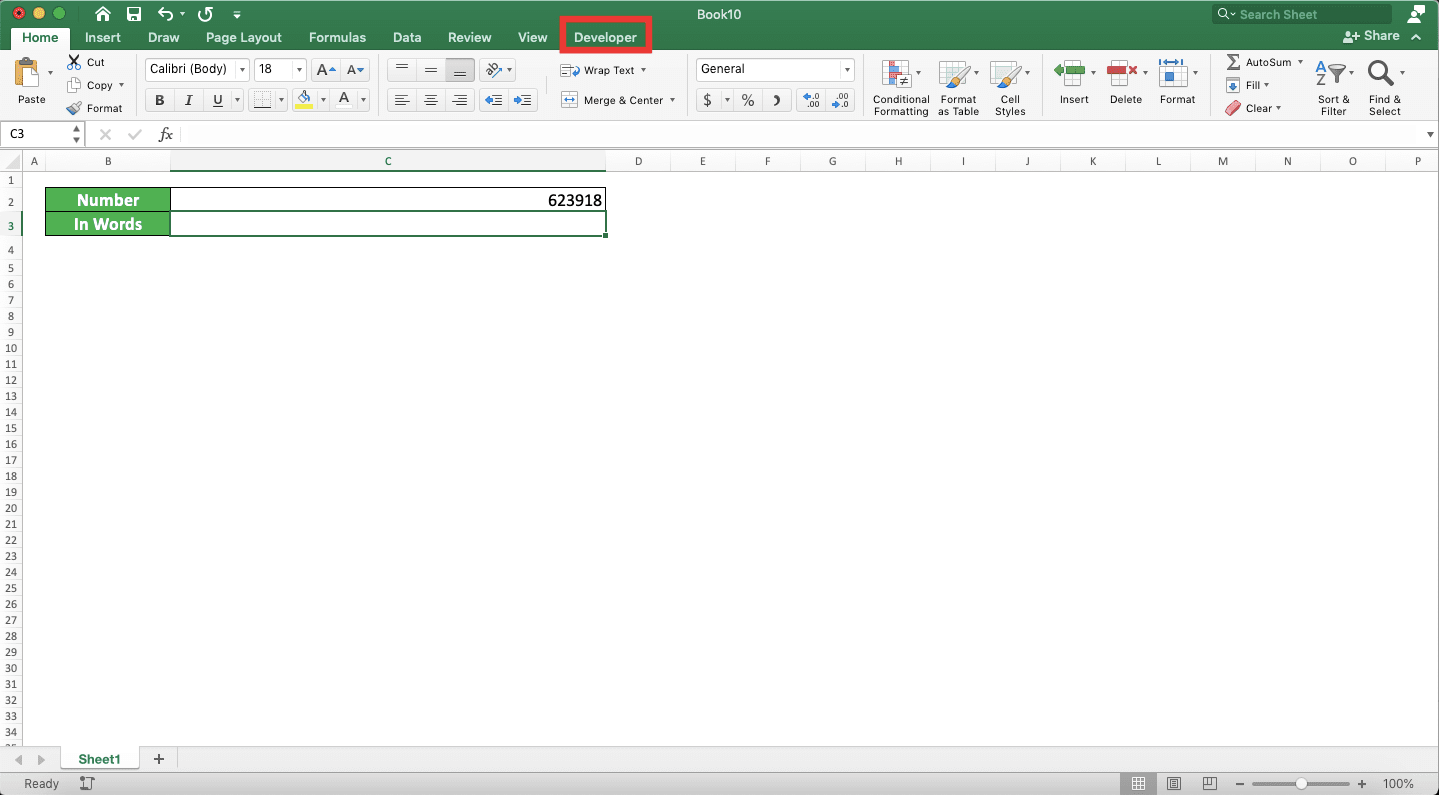2. Click the Excel Add-Ins button3. In the dialog box that shows up, browse to where the add-in file you download is to open it
4. Check the checkbox of the Numbertotext add-in and click OK5. Done! Now, you can use the NUMBERTOTEXT formula to convert your number into words!## How to Convert Number to Words with Currency in Excel

As number conversion to words is usually done for financial numbers, you may need to add currency in the conversion result. For that, you can use CONCATENATE, CONCAT, or the ampersand symbol (&) to add the currency word.

For example, if you use the NUMBERTOTEXT custom formula to convert your number, then the writing will be like this. This assumes you want to add the “dollars” currency word behind the conversion result. We use the ampersand symbol to add the currency word for this.

= NUMBERTOTEXT ( number ) & “ dollars”

Don’t forget to add a space in front of the “dollars” word.

Here is the implementation example of this method in excel.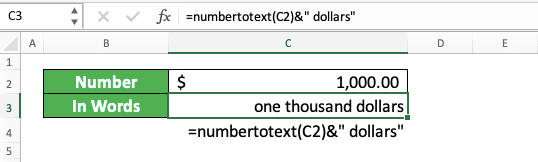Quite easy, isn’t it?

## How to Capitalize Each First Letter in the Result from Converting a Number to Words in Excel: PROPER

If you need to capitalize the first letter of each word from the number conversion, then you need a specific formula. That formula is PROPER.

You can envelop the number conversion formula you use with PROPER to do the capitalization. Generally, here is the general writing form of that, assuming you use the NUMBERTOTEXT formula.

= PROPER ( NUMBERTOTEXT ( number ) )

Simple, isn’t it? And here is the example of the PROPER usage for the conversion result.## How to Capitalize All Letters in the Result from Converting a Number to Words in Excel: UPPER

What about if we want to capitalize all the letters instead? For that, you should use UPPER!

The way to use UPPER here is the same as the way we use PROPER earlier. Here is the general writing form of the UPPER implementation to our NUMBERTOTEXT formula.

= UPPER ( NUMBERTOTEXT ( number ) )

And here is the implementation example of that writing.## Exercise

After you have learned how to convert numbers to words in excel using various methods, let’s do an exercise! We make this exercise so you can understand more practically about this tutorial topic.

### Instruction:

Use whichever method you prefer to convert each number to words in the provided cells for the answers. Do it while keeping these requirements.
1. Convert the number into words
2. Convert the number into words with the currency word (US dollars)
3. Convert the number into words with the currency word (US dollars) and capitalize all the letters of the conversion result

• The NUMBERTOTEXT formula cannot be used to convert decimal digits you have in your number to words
• If you input the VBA code manually, then you won’t find the NUMBERTOTEXT formula if you create a new workbook. You must use the add-in for that or you must paste the VBA code to create the NUMBERTOTEXT formula again

Related tutorials you should learn too:

Get updated excel info from Compute Expert by registering your email. It's free!